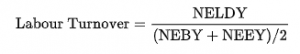# Calculation of Employee Turnover

Labour turnover is equal to the number of employees leaving, divided by the average total number of employees (in order to give a percentage value). The number of employees leaving and the total number of employees are measured over one calendar year.Where:
NELDY = Number of Employees who Left During the Year
NEBY = Number of Employees at the Beginning of the Year
NEEY = Number of Employees at the End of the Year

For example, at the start of the year a business had 40 employees, but during the year 9 staff resigned with 2 new hires, thus leaving 33 staff members at the end of the year. Hence this year’s turnover is 25%. This is derived from, (9/((40+33)/2)) = 25%. However the above formula should be applied with caution if data is grouped. For example, if attrition rate is calculated for Employees with tenure 1 to 4 years, above formula may result artificially inflated attrition rate as employees with tenure more than 4 years are not counted in the denominator. -wikipedia